# NCERT Exemplar Class 10 Maths Solutions for Chapter 4 - Quadratic Equations

## NCERT Exemplar Solutions Class 10 Maths Chapter 4 – Free PDF Download

NCERT Exemplar Solutions Class 10 Maths Chapter 4 Quadratic Equations are provided here to help students prepare for the board exams. The exemplars have been prepared by subject experts in accordance with the latest CBSE syllabus (2023-2024) and are available in PDF, which can be downloaded easily.

Chapter 4 in Class 10 Maths is an important chapter for Class 10 students. Besides, there will be several questions based on this chapter, and students have to solve these problems using quadratic equations. Further, while dealing with this chapter, students will learn about different quadratic equations and how to find solutions by either using the factorisation method or the square method. To help students grasp the complete concepts of this chapter, free NCERT Exemplar for quadratic equations is provided here. Click here to get exemplars for all chapters.

Students can go through the exemplar problems and solutions for Chapter 4 to understand the concepts introduced in this chapter, such as:

• Representing the given situations mathematically in the form of quadratic equations
• Solving quadratic equations with the help of the factorisation method
• Solving quadratic equations by completing the square
• Determining the nature of roots

Students can access the Class 10 Maths Chapter 4 NCERT Exemplar PDF below.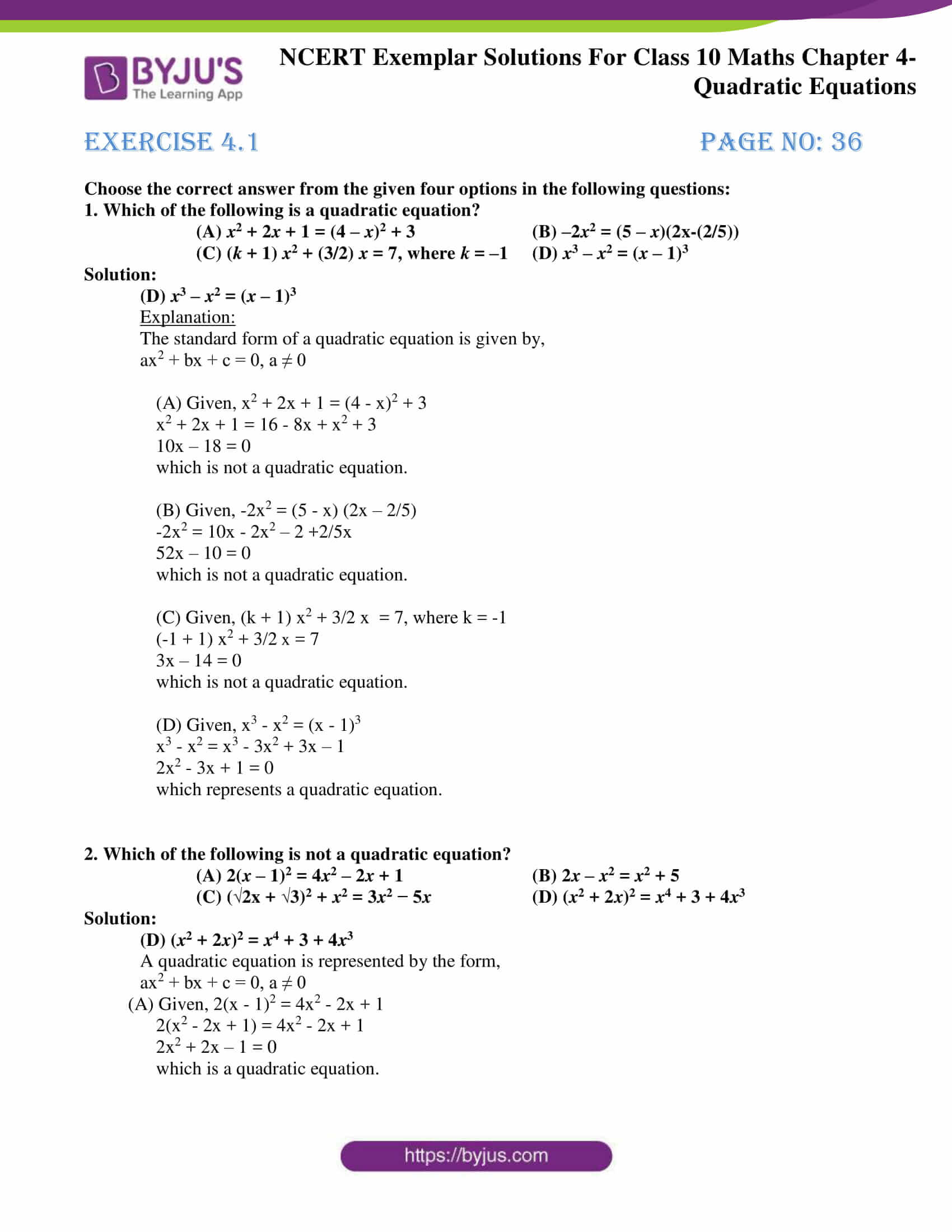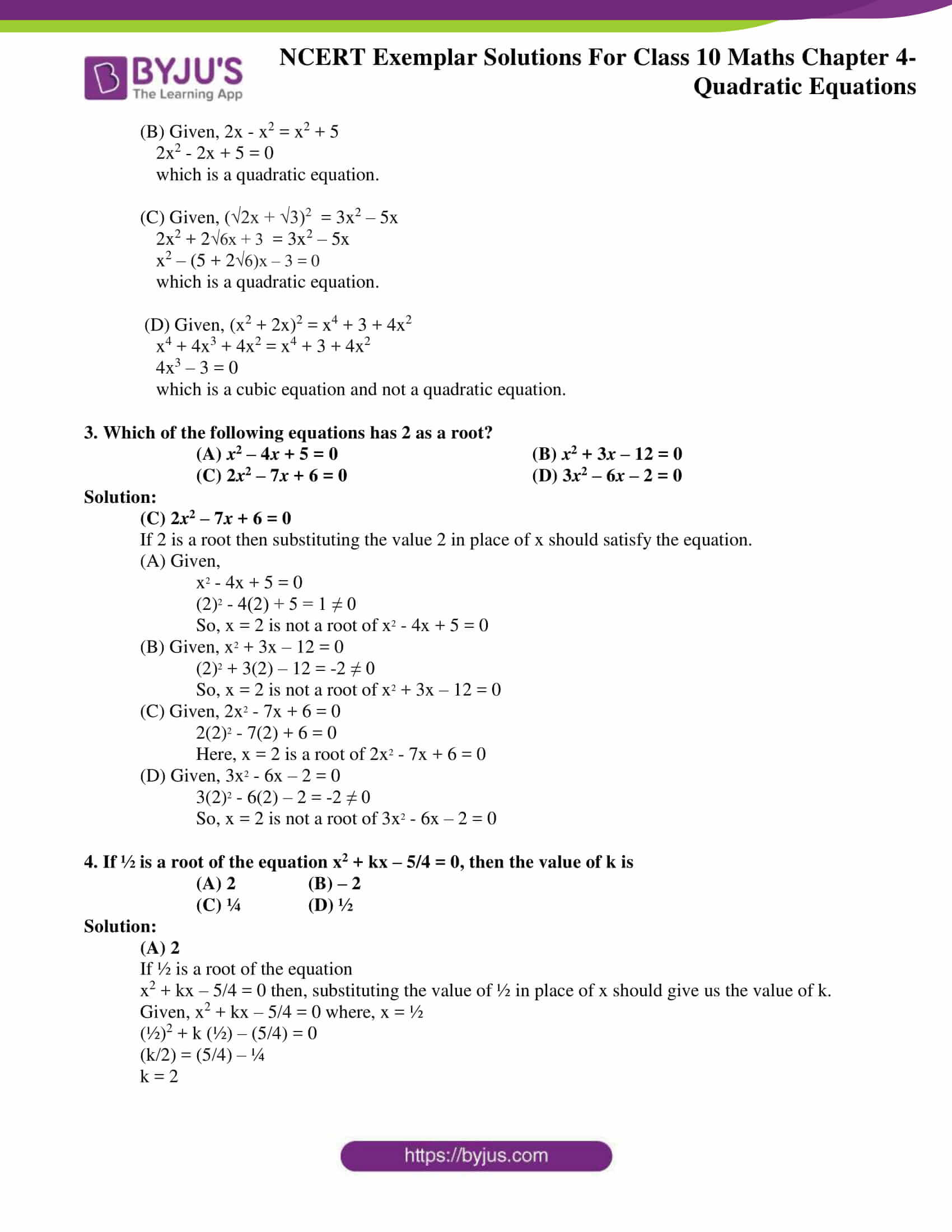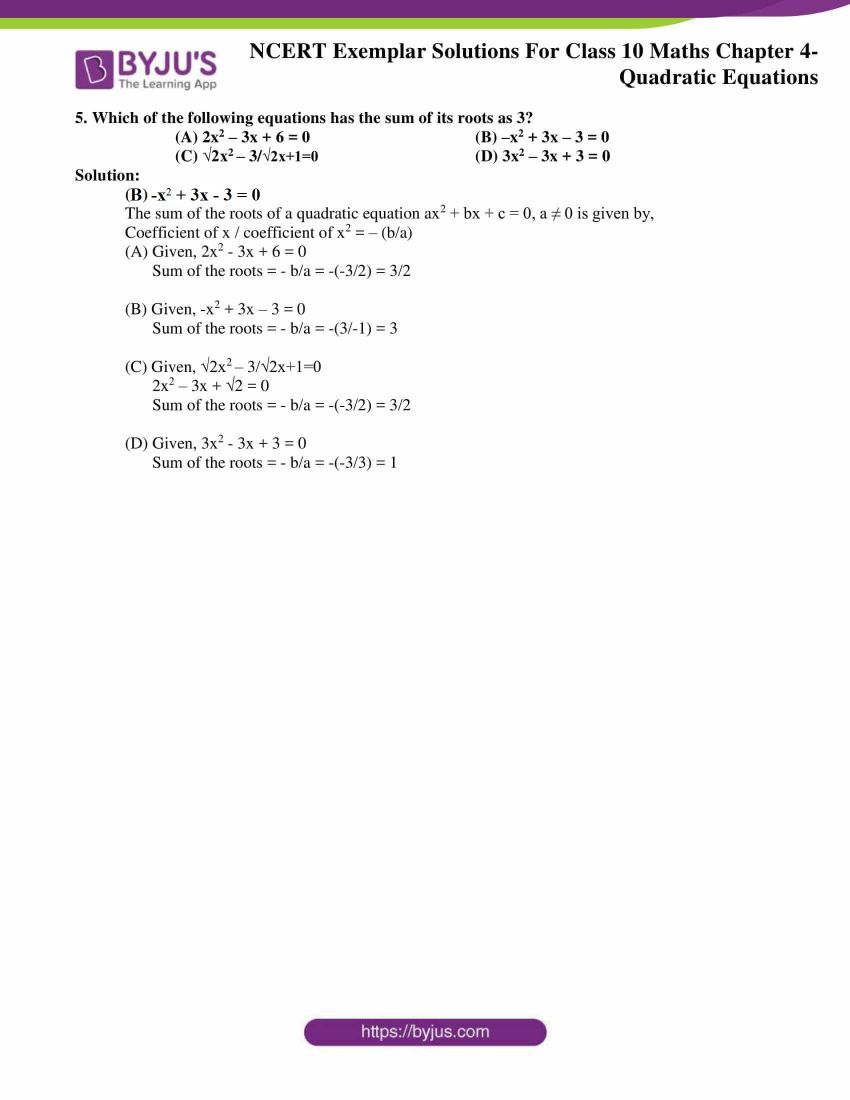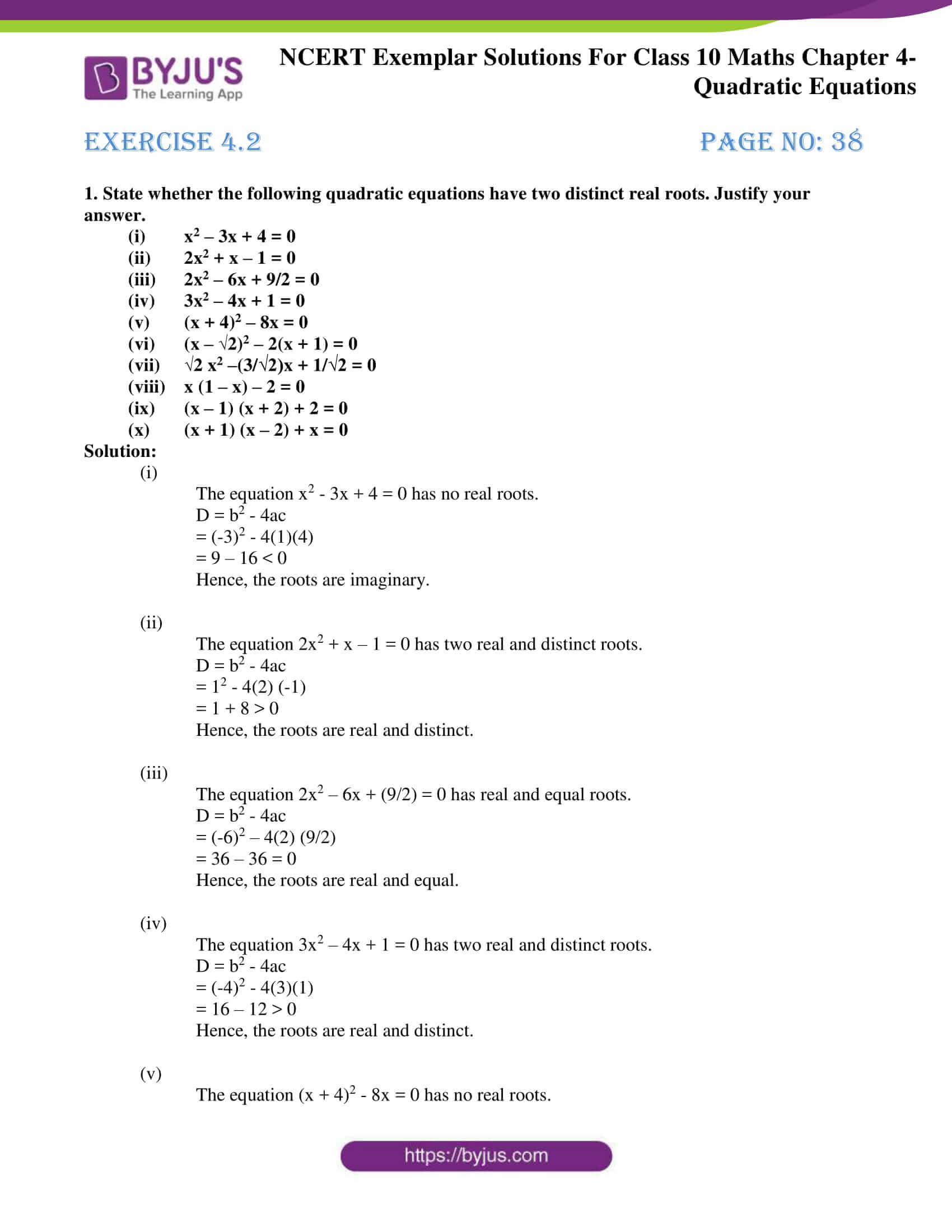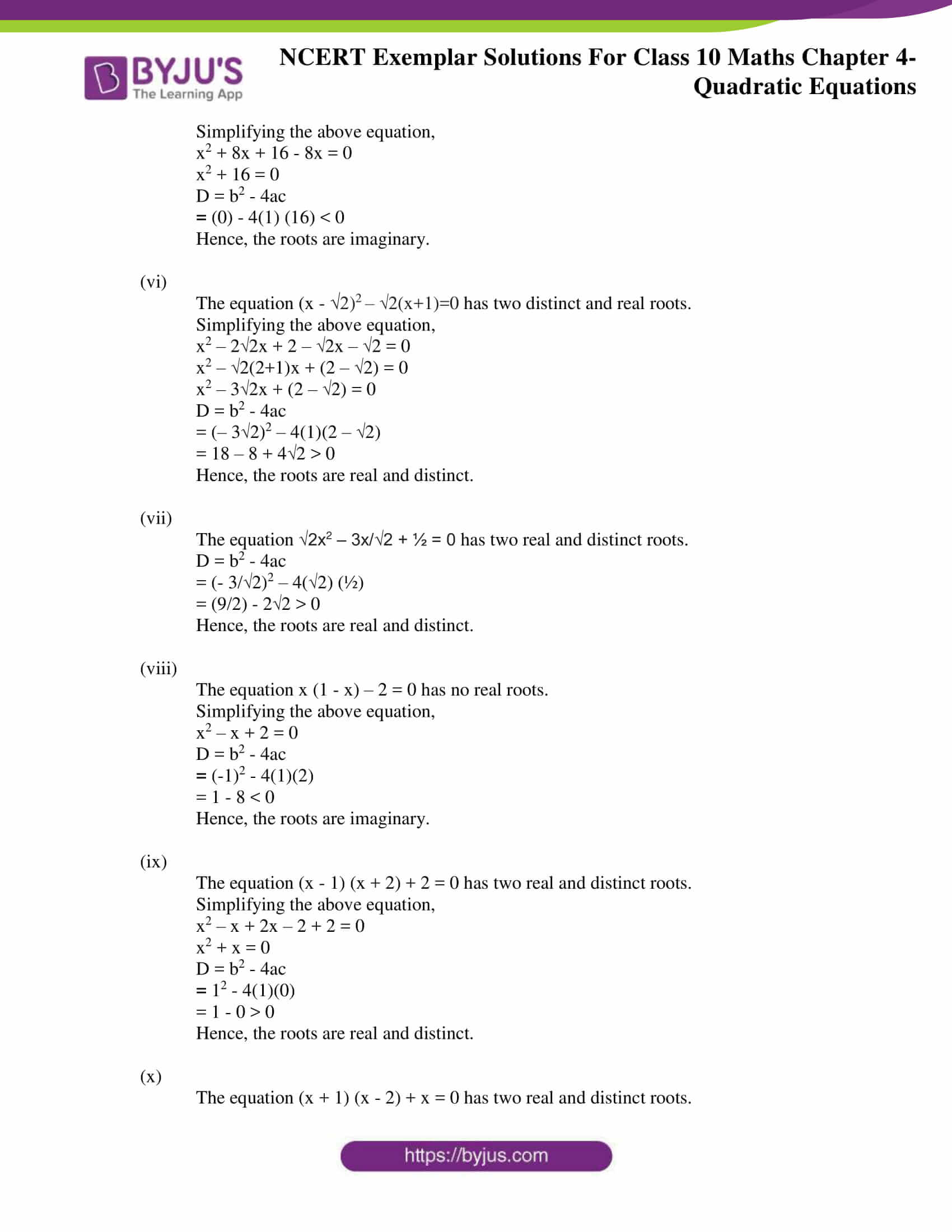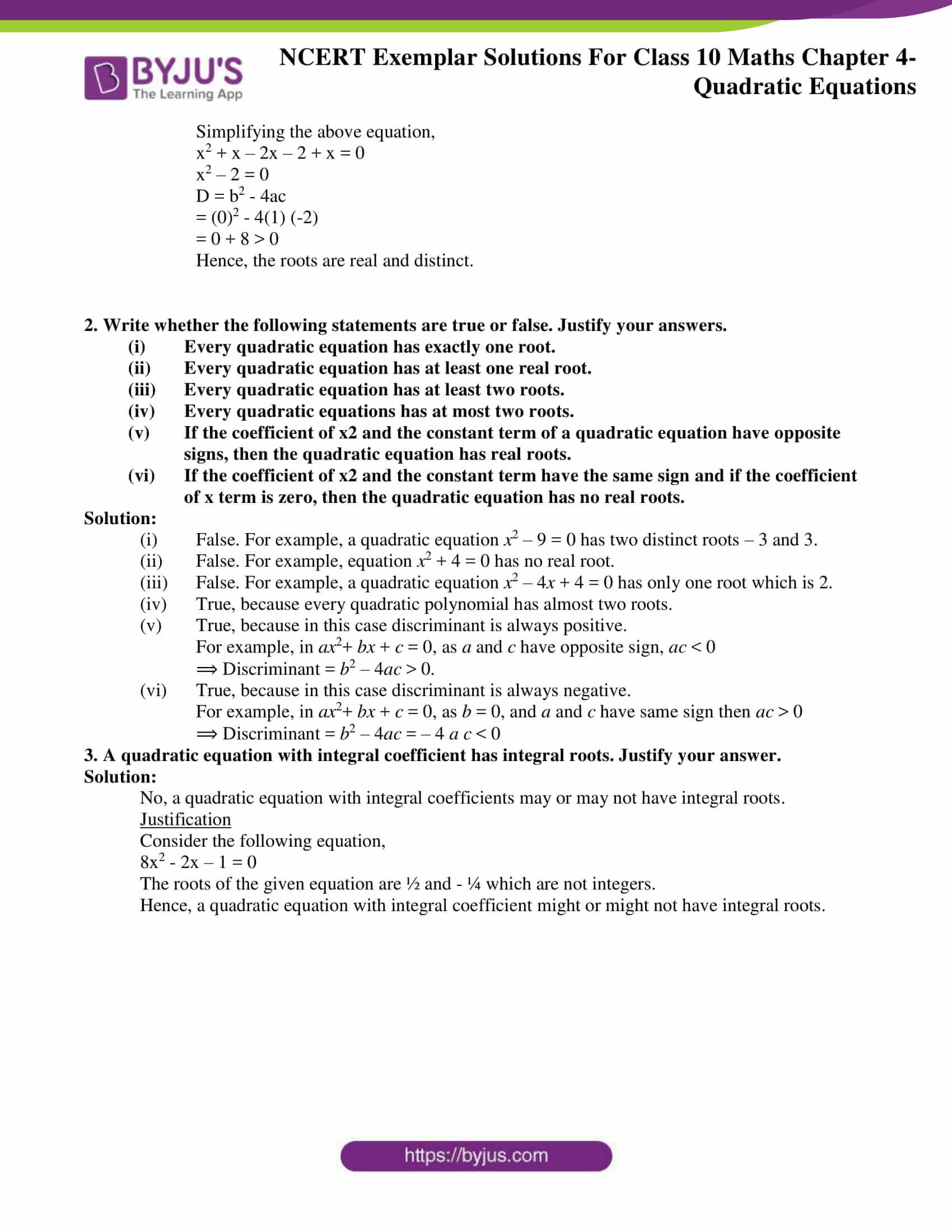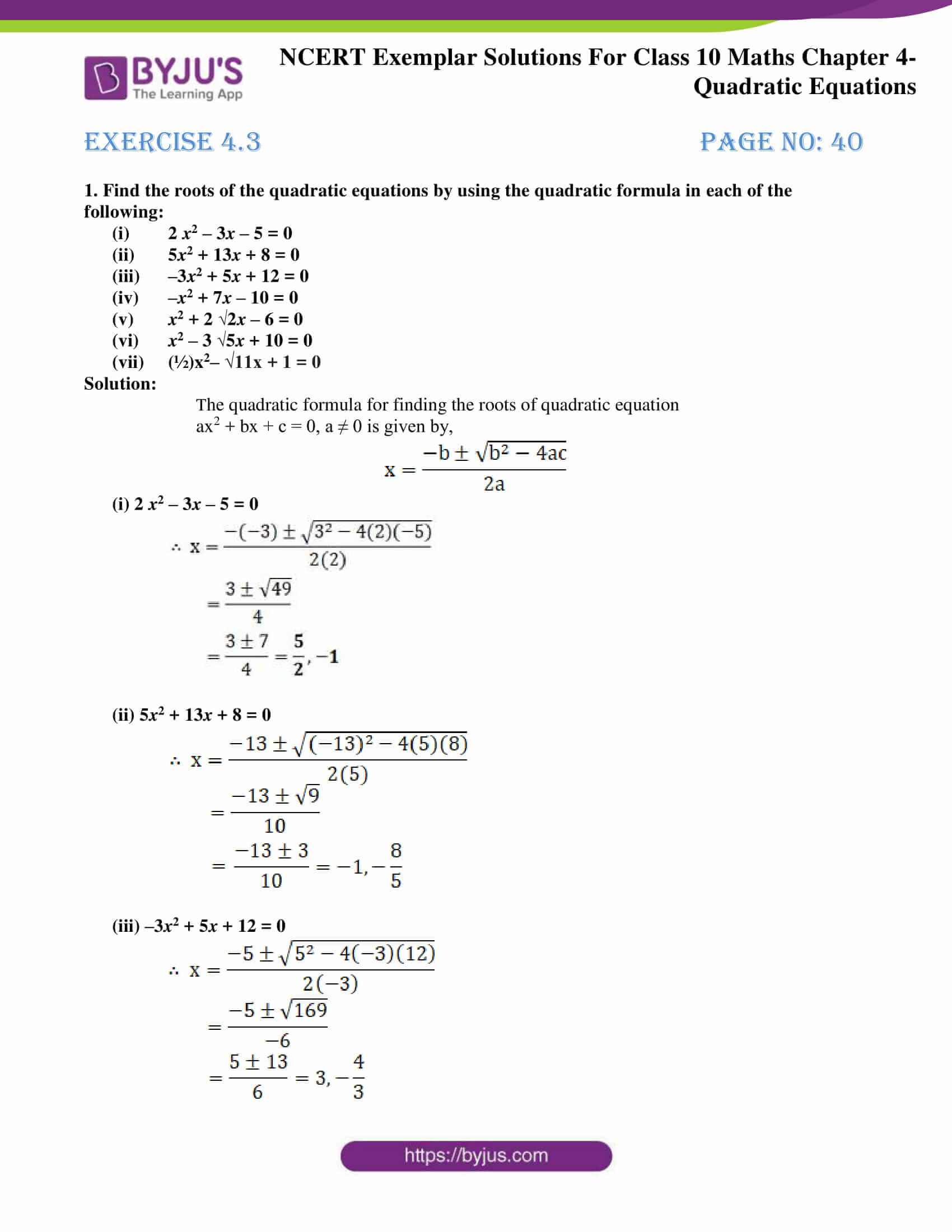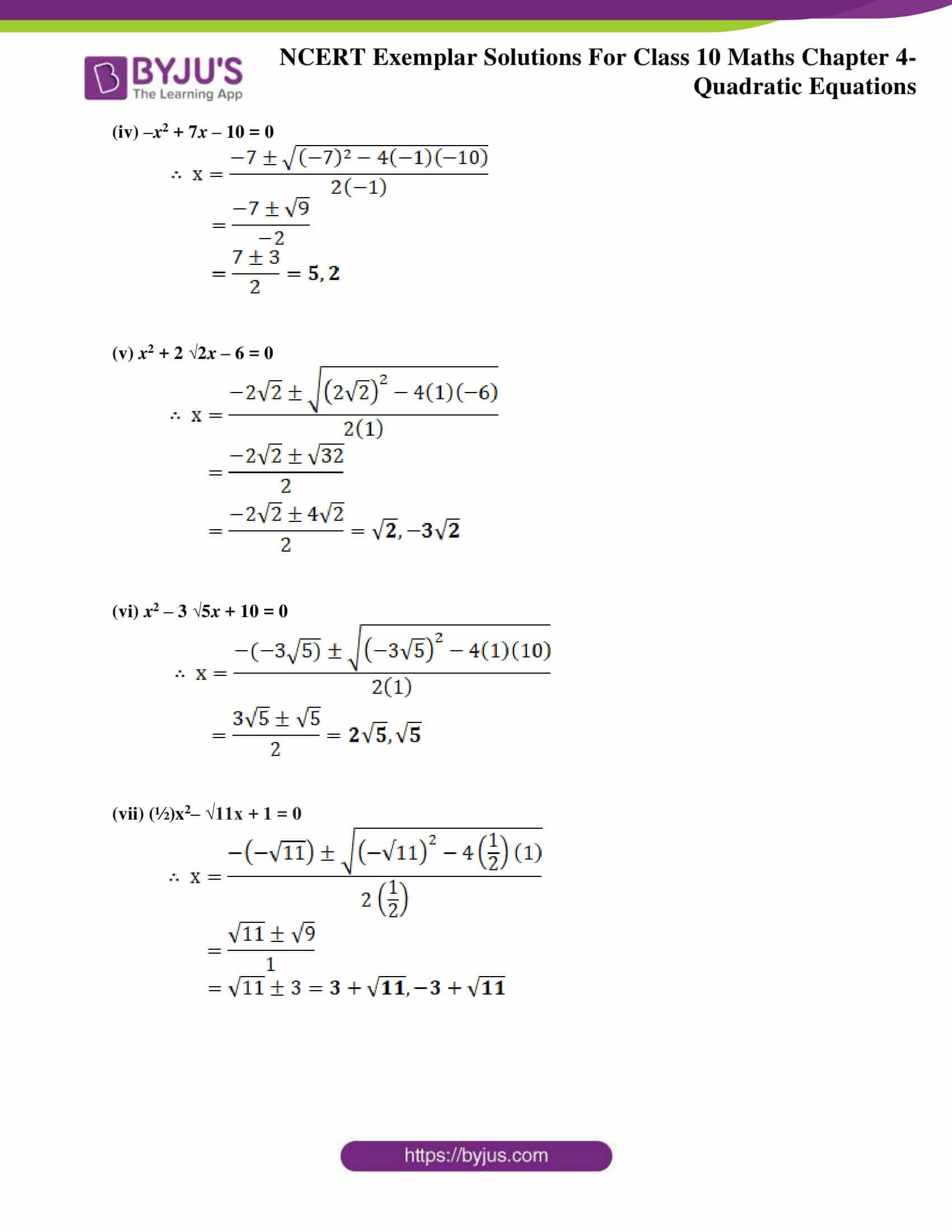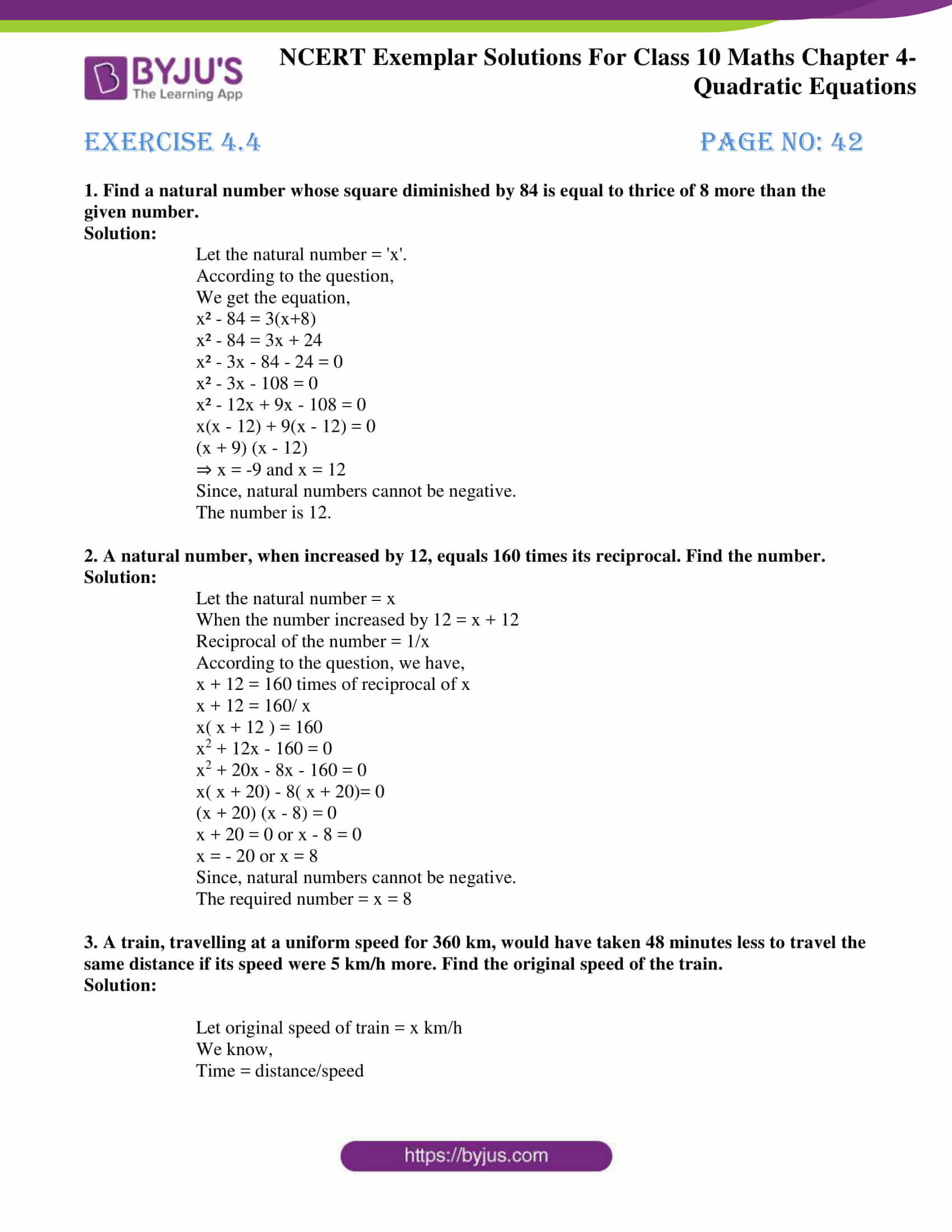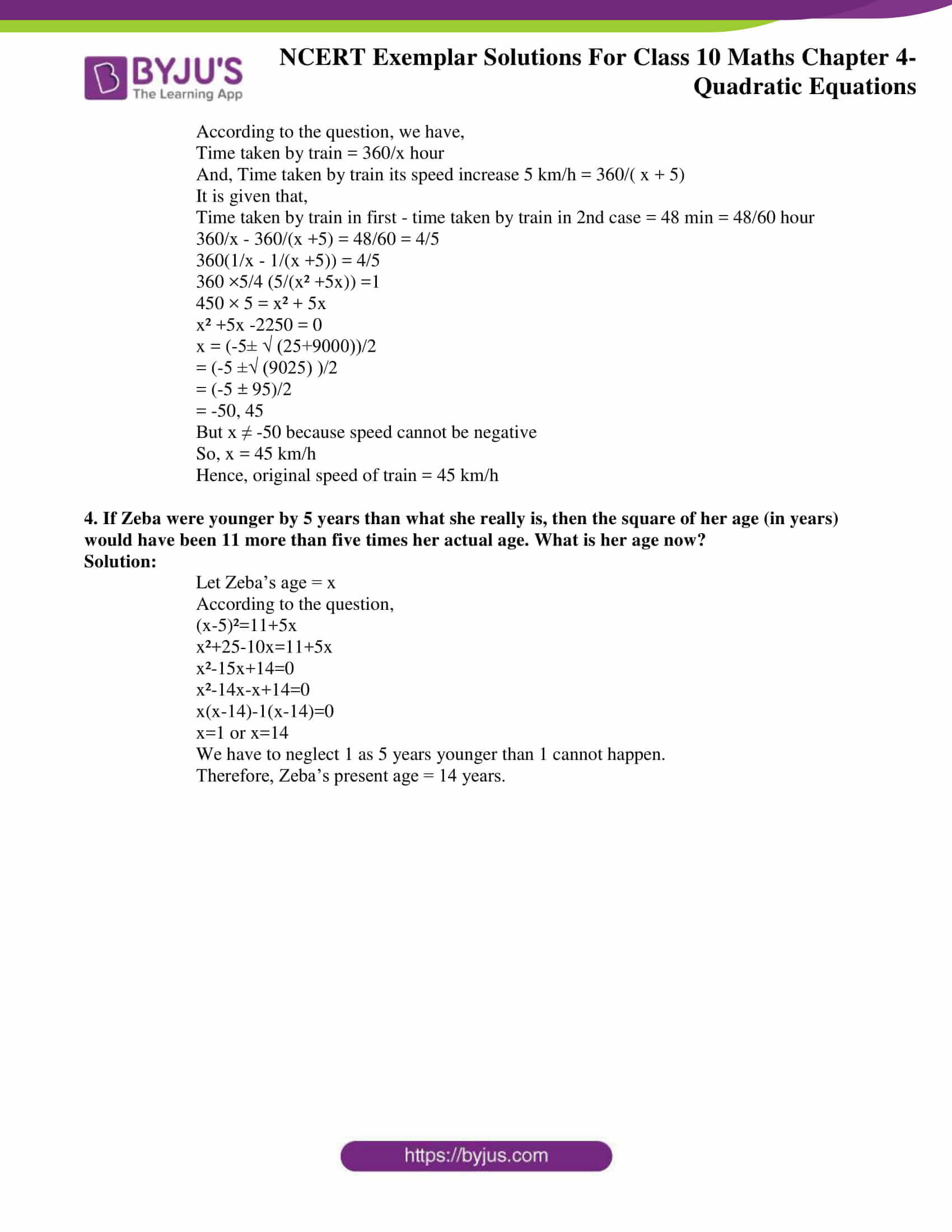## Exercise 4.1

Choose the correct answer from the given four options in the following questions:

1. Which of the following is a quadratic equation?

(A) x2 + 2x + 1 = (4 – x)2 + 3 (B) –2x2 = (5 – x)(2x-(2/5))

(C) (k + 1) x2 + (3/2) x = 7, where k = –1 (D) x3x2 = (x – 1)3

Solution:

(D) x3x2 = (x – 1)3

Explanation:

The standard form of a quadratic equation is given by,

ax2 + bx + c = 0, a ≠ 0

(A) Given, x2 + 2x + 1 = (4 – x)2 + 3

x2 + 2x + 1 = 16 – 8x + x2 + 3

10x – 18 = 0

which is not a quadratic equation.

(B) Given, -2x2 = (5 – x) (2x – 2/5)

-2x2 = 10x – 2x2 – 2 +2/5x

52x – 10 = 0

which is not a quadratic equation.

(C) Given, (k + 1) x2 + 3/2 x  = 7, where k = -1

(-1 + 1) x2 + 3/2 x = 7

3x – 14 = 0

which is not a quadratic equation.

(D) Given, x3 – x2 = (x – 1)3

x3 – x2 = x3 – 3x2 + 3x – 1

2x2 – 3x + 1 = 0

2. Which of the following is not a quadratic equation?

(A) 2(x – 1)2 = 4x2 – 2x + 1 (B) 2x x2 = x2 + 5

(C) (2x + 3)2 + x2 = 3x2 − 5x (D) (x2 + 2x)2 = x4 + 3 + 4x3

Solution:

(D) (x2 + 2x)2 = x4 + 3 + 4x3

A quadratic equation is represented by the form,

ax2 + bx + c = 0, a ≠ 0

(A) Given, 2(x – 1)2 = 4x2 – 2x + 1

2(x2 – 2x + 1) = 4x2 – 2x + 1

2x2 + 2x – 1 = 0

(B) Given, 2x – x2 = x2 + 5

2x2 – 2x + 5 = 0

(C) Given, (√2x + √3)2  = 3x2 – 5x

2x2 + 2√6x + 3  = 3x2 – 5x

x2 – (5 + 2√6)x – 3 = 0

(D) Given, (x2 + 2x)2 = x4 + 3 + 4x2

x4 + 4x3 + 4x2 = x4 + 3 + 4x2

4x3 – 3 = 0

which is a cubic equation and not a quadratic equation.

3. Which of the following equations has 2 as a root?

(A) x2 – 4x + 5 = 0 (B) x2 + 3x – 12 = 0

(C) 2x2 – 7x + 6 = 0 (D) 3x2 – 6x – 2 = 0

Solution:

(C) 2x2 – 7x + 6 = 0

If 2 is a root then substituting the value 2 in place of x should satisfy the equation.

(A) Given,

x2 – 4x + 5 = 0

(2)2 – 4(2) + 5 = 1 ≠ 0

So, x = 2 is not a root of x2 – 4x + 5 = 0

(B) Given, x2 + 3x – 12 = 0

(2)2 + 3(2) – 12 = -2 ≠ 0

So, x = 2 is not a root of x2 + 3x – 12 = 0

(C) Given, 2x2 – 7x + 6 = 0

2(2)2 – 7(2) + 6 = 0

Here, x = 2 is a root of 2x2 – 7x + 6 = 0

(D) Given, 3x2 – 6x – 2 = 0

3(2)2 – 6(2) – 2 = -2 ≠ 0

So, x = 2 is not a root of 3x2 – 6x – 2 = 0

4. If ½ is a root of the equation x2 + kx – 5/4 = 0, then the value of k is

(A) 2 (B) – 2

(C) ¼ (D) ½

Solution:

(A) 2

If ½ is a root of the equation

x2 + kx – 5/4 = 0 then, substituting the value of ½ in place of x should give us the value of k.

Given, x2 + kx – 5/4 = 0 where, x = ½

(½)2 + k (½) – (5/4) = 0

(k/2) = (5/4) – ¼

k = 2

5. Which of the following equations has the sum of its roots as 3?

(A) 2x2 – 3x + 6 = 0 (B) –x2 + 3x – 3 = 0

(C) √2x2 – 3/√2x+1=0 (D) 3x2 – 3x + 3 = 0

Solution:

(B) –x2 + 3x – 3 = 0

The sum of the roots of a quadratic equation ax2 + bx + c = 0, a ≠ 0 is given by,

Coefficient of x / coefficient of x2 = – (b/a)

(A) Given, 2x2 – 3x + 6 = 0

Sum of the roots = – b/a = -(-3/2) = 3/2

(B) Given, -x2 + 3x – 3 = 0

Sum of the roots = – b/a = -(3/-1) = 3

(C) Given, √2x2 – 3/√2x+1=0

2x2 – 3x + √2 = 0

Sum of the roots = – b/a = -(-3/2) = 3/2

(D) Given, 3x2 – 3x + 3 = 0

Sum of the roots = – b/a = -(-3/3) = 1

## Exercise 4.2

1. x2 – 3x + 4 = 0
2. 2x2 + x – 1 = 0
3. 2x2 – 6x + 9/2 = 0
4. 3x2 – 4x + 1 = 0
5. (x + 4)2 – 8x = 0
6. (x – 2)2 – 2(x + 1) = 0
7. 2 x2 –(3/√2)x + 1/√2 = 0
8. x (1 – x) – 2 = 0
9. (x – 1) (x + 2) + 2 = 0
10. (x + 1) (x – 2) + x = 0

Solution:

(i)

The equation x2 – 3x + 4 = 0 has no real roots.

D = b2 – 4ac

= (-3)2 – 4(1)(4)

= 9 – 16 < 0

Hence, the roots are imaginary.

(ii)

The equation 2x2 + x – 1 = 0 has two real and distinct roots.

D = b2 – 4ac

= 12 – 4(2) (-1)

= 1 + 8 > 0

Hence, the roots are real and distinct.

(iii)

The equation 2x2 – 6x + (9/2) = 0 has real and equal roots.

D = b2 – 4ac

= (-6)2 – 4(2) (9/2)

= 36 – 36 = 0

Hence, the roots are real and equal.

(iv)

The equation 3x2 – 4x + 1 = 0 has two real and distinct roots.

D = b2 – 4ac

= (-4)2 – 4(3)(1)

= 16 – 12 > 0

Hence, the roots are real and distinct.

(v)

The equation (x + 4)2 – 8x = 0 has no real roots.

Simplifying the above equation,

x2 + 8x + 16 – 8x = 0

x2 + 16 = 0

D = b2 – 4ac

= (0) – 4(1) (16) < 0

Hence, the roots are imaginary.

(vi)

The equation (x – √2)2 – √2(x+1)=0 has two distinct and real roots.

Simplifying the above equation,

x2 – 2√2x + 2 – √2x – √2 = 0

x2 – √2(2+1)x + (2 – √2) = 0

x2 – 3√2x + (2 – √2) = 0

D = b2 – 4ac

= (– 3√2)2 – 4(1)(2 – √2)

= 18 – 8 + 4√2 > 0

Hence, the roots are real and distinct.

(vii)

The equation √2x2 – 3x/√2 + ½ = 0 has two real and distinct roots.

D = b2 – 4ac

= (- 3/√2)2 – 4(√2) (½)

= (9/2) – 2√2 > 0

Hence, the roots are real and distinct.

(viii)

The equation x (1 – x) – 2 = 0 has no real roots.

Simplifying the above equation,

x2 – x + 2 = 0

D = b2 – 4ac

= (-1)2 – 4(1)(2)

= 1 – 8 < 0

Hence, the roots are imaginary.

(ix)

The equation (x – 1) (x + 2) + 2 = 0 has two real and distinct roots.

Simplifying the above equation,

x2 – x + 2x – 2 + 2 = 0

x2 + x = 0

D = b2 – 4ac

= 12 – 4(1)(0)

= 1 – 0 > 0

Hence, the roots are real and distinct.

(x)

The equation (x + 1) (x – 2) + x = 0 has two real and distinct roots.

Simplifying the above equation,

x2 + x – 2x – 2 + x = 0

x2 – 2 = 0

D = b2 – 4ac

= (0)2 – 4(1) (-2)

= 0 + 8 > 0

Hence, the roots are real and distinct.

2. Write whether the following statements are true or false. Justify your answers.

1. Every quadratic equation has exactly one root.
2. Every quadratic equation has at least one real root.
3. Every quadratic equation has at least two roots.
4. Every quadratic equations has at most two roots.
5. If the coefficient of x2 and the constant term of a quadratic equation have opposite signs, then the quadratic equation has real roots.
6. If the coefficient of x2 and the constant term have the same sign and if the coefficient of x term is zero, then the quadratic equation has no real roots.

Solution:

(i) False. For example, a quadratic equation x2 – 9 = 0 has two distinct roots – 3 and 3.

(ii) False. For example, equation x2 + 4 = 0 has no real root.

(iii) False. For example, a quadratic equation x2 – 4+ 4 = 0 has only one root which is 2.

(iv) True, because every quadratic polynomial has almost two roots.

(v) True, because in this case discriminant is always positive.

For example, in ax2bx + c = 0, as a and c have opposite sign, ac < 0

⟹ Discriminant = b2 – 4ac > 0.

(vi) True, because in this case discriminant is always negative.

For example, in ax2bx + c = 0, as b = 0, and a and c have same sign then ac > 0

⟹ Discriminant = b2 – 4ac = – 4 a c < 0

Solution:

No, a quadratic equation with integral coefficients may or may not have integral roots.

Justification

Consider the following equation,

8x2 – 2x – 1 = 0

The roots of the given equation are ½ and – ¼ which are not integers.

Hence, a quadratic equation with integral coefficient might or might not have integral roots.

## Exercise 4.3

1. Find the roots of the quadratic equations by using the quadratic formula in each of the following:

1. 2 x2 – 3x – 5 = 0
2. 5x2 + 13x + 8 = 0
3. –3x2 + 5x + 12 = 0
4. x2 + 7x – 10 = 0
5. x2 + 22x – 6 = 0
6. x2 – 35x + 10 = 0
7. (½)x2– √11x + 1 = 0

Solution:

ax2 + bx + c = 0, a ≠ 0 is given by,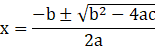(i) 2 x2 – 3x – 5 = 0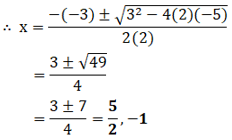(ii) 5x2 + 13x + 8 = 0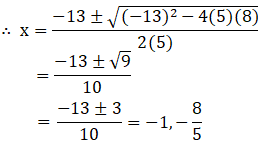(iii) –3x2 + 5x + 12 = 0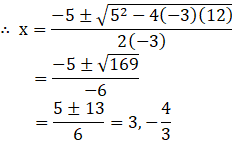(iv) –x2 + 7x – 10 = 0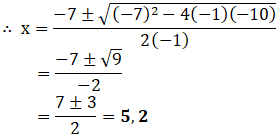(v) x2 + 22x – 6 = 0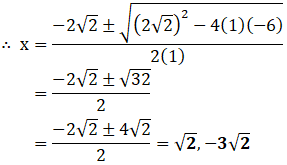(vi) x2 – 35x + 10 = 0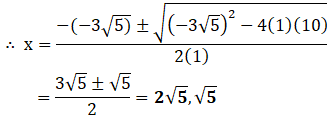(vii) (½)x2– √11x + 1 = 0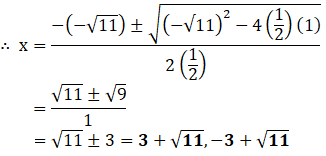## Exercise 4.4

1. Find a natural number whose square diminished by 84 is equal to thrice of 8 more than the given number.

Solution:

Let the natural number = ‘x’.

According to the question,

We get the equation,

x² – 84 = 3(x+8)

x² – 84 = 3x + 24

x² – 3x – 84 – 24 = 0

x² – 3x – 108 = 0

x² – 12x + 9x – 108 = 0

x(x – 12) + 9(x – 12) = 0

(x + 9) (x – 12)

⇒ x = -9 and x = 12

Since, natural numbers cannot be negative.

The number is 12.

2. A natural number, when increased by 12, equals 160 times its reciprocal. Find the number.

Solution:

Let the natural number = x

When the number increased by 12 = x + 12

Reciprocal of the number = 1/x

According to the question, we have,

x + 12 = 160 times of reciprocal of x

x + 12 = 160/ x

x( x + 12 ) = 160

x2 + 12x – 160 = 0

x2 + 20x – 8x – 160 = 0

x( x + 20) – 8( x + 20)= 0

(x + 20) (x – 8) = 0

x + 20 = 0 or x – 8 = 0

x = – 20 or x = 8

Since, natural numbers cannot be negative.

The required number = x = 8

3. A train, travelling at a uniform speed for 360 km, would have taken 48 minutes less to travel the same distance if its speed were 5 km/h more. Find the original speed of the train.

Solution:

Let original speed of train = x km/h

We know,

Time = distance/speed

According to the question, we have,

Time taken by train = 360/x hour

And, Time taken by train its speed increase 5 km/h = 360/( x + 5)

It is given that,

Time taken by train in first – time taken by train in 2nd case = 48 min = 48/60 hour

360/x – 360/(x +5) = 48/60 = 4/5

360(1/x – 1/(x +5)) = 4/5

360 ×5/4 (5/(x² +5x)) =1

450 × 5 = x² + 5x

x² +5x -2250 = 0

x = (-5± √ (25+9000))/2

= (-5 ±√ (9025) )/2

= (-5 ± 95)/2

= -50, 45

But x ≠ -50 because speed cannot be negative

So, x = 45 km/h

Hence, original speed of train = 45 km/h

4. If Zeba were younger by 5 years than what she really is, then the square of her age (in years) would have been 11 more than five times her actual age. What is her age now?

Solution:

Let Zeba’s age = x

According to the question,

(x-5)²=11+5x

x²+25-10x=11+5x

x²-15x+14=0

x²-14x-x+14=0

x(x-14)-1(x-14)=0

x=1 or x=14

We have to neglect 1 as 5 years younger than 1 cannot happen.

Therefore, Zeba’s present age = 14 years.

BYJU’S provides online study materials such as notes, exemplar books, question papers, and Maths NCERT Solutions for Class 10 to help students prepare for board exams in the most efficient way and score good marks. All these materials are available in downloadable PDFs. Students can also get an idea of the question pattern and marking scheme of Chapter 4 in the board exam by solving the sample papers and previous years’ question papers.

These exemplars will help students to solve problems in the right way as well as find answers to the most difficult questions given at the end of the chapter. Students can use these solved questions as a reference tool to practise Maths effectively and, in the process, develop good Math skills. They can also prepare for competitive exams using these exemplar solutions.

Get updated study materials to learn from us, and also download BYJU’S – The Learning App to experience a new method of learning with the help of educational videos clearing the concepts of Maths topics such as quadratic equations, linear equations, etc., in a visual way.

## Frequently Asked Questions on NCERT Exemplar Solutions for Class 10 Maths Chapter 4

Q1

### How many problems are there in NCERT Exemplar Solutions for Class 10 Maths Chapter 4?

Exercise 4.1 of NCERT Exemplar Solutions has 5 questions, Exercise 4.2 has 3 questions, Exercise 4.3 has 1 question with many sub-questions, and Exercise 4.4 has 4 questions. Long answers, short answers and MCQs are present in each exercise, covering the topics which are important from the exam point of view. The solutions for the exercise-wise problems are designed by a team of subject experts at BYJU’S, having vast conceptual knowledge.
Q2

### What are the topics covered under NCERT Exemplar Solutions for Class 10 Maths Chapter 4?

Students can go through the exemplar problems and solutions for Chapter 4 to understand the concepts introduced in this chapter, such as:
1. Representing the given situations mathematically in the form of quadratic equations
2. Solving quadratic equations with the help of the factorisation method
3. Solving quadratic equations by completing the square
4. Determining the nature of roots
Q3

### What are the roots of quadratic equations according to NCERT Exemplar Solutions for Class 10 Maths Chapter 4?

The values of variables satisfying the given quadratic equation are called their roots. In other words, x = α is the root of the quadratic equation f(x) if f(α) = 0.
The real roots of equation f(x) = 0 are the x-coordinates of the points where the curve y = f(x) intersects the x-axis.
1. One of the roots of the quadratic equation is zero, and the other is -b/a if c = 0
2. Both the roots are zero if b = c = 0
3. The roots are reciprocal to each other if a = c